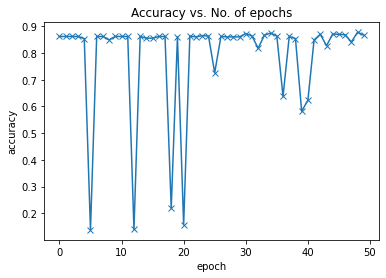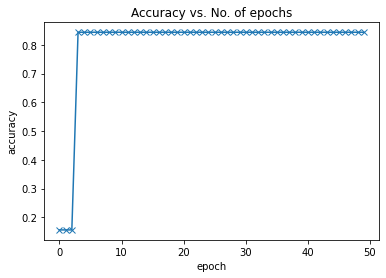# Model is not converging in case of custom transformer based learning

My code for a custom model based on the transformer encoder layer of the Vision Transformer is not converging with the binary classification task as shown below, while the multi-class classification is converging with 50 epochs and SGD optimizer with a learning rate of 0.005. I tried with both cross-entropy and binary cross-entropy loss functions, but both didn’t work. What might be the issues here?The code for the model is as follows

``````class ViT(ImageClassificationBase):
def __init__(self,
img_size=224, # from Table 3
num_channels=3,
patch_size=16,
embedding_dim=768, # from Table 1
dropout=0.1,

num_transformer_layers=6, # from Table 1
num_classes=2)
super().__init__()
self.layers = num_transformer_layers
# Assert image size is divisible by patch size
assert img_size % patch_size == 0, "Image size must be divisble by patch size."
assert embedding_dim % num_heads == 0, "Embedding dimension should be divisible by num_heads."

# 1. Create patch embedding
self.patch_embedding = PatchEmbedding(in_channels=num_channels,
patch_size=patch_size,
embedding_dim=embedding_dim)

# 2. Create class token
self.class_token = nn.Parameter(torch.randn(1, 1, embedding_dim),

# 3. Create positional embedding
num_patches = (img_size * img_size) // patch_size**2 # N = HW/P^2
self.positional_embedding = nn.Parameter(torch.randn(1, num_patches+1, embedding_dim))

self.embedding_dropout = nn.Dropout(p=dropout)

nn.LayerNorm(normalized_shape=embedding_dim),
nn.Linear(in_features=embedding_dim,
out_features=num_classes)
)

def forward(self, x):

batch_size = x.shape
# Create the patch embedding
x = self.patch_embedding(x)

init_patch = x

# First, expand the class token across the batch size
class_token = self.class_token.expand(batch_size, -1, -1)

# Prepend the class token to the patch embedding
x = torch.cat((class_token, x), dim=1)

# Add the positional embedding to patch embedding with class token
x = self.positional_embedding + x

# Dropout on patch + positional embedding
x = self.embedding_dropout(x)

# Pass embedding through Transformer Encoder stack
for l in range(self.layers):

encoder_feat = self.transformer_encoder_layer(x)

x = encoder_feat[:,1:]+init_patch
x= torch.cat((encoder_feat[:,0].unsqueeze(1),x),dim=1)

# Pass 0th index of x through MLP head

return x
``````

Try to overfit a small dataset split (e.g. just 10 samples) by playing around with hyperparameters. Once this is done you could scale up the use case again. If your model isn’t able to overfit 10 samples, you might have some errors in your code or the architecture is not suitable for the use case.

1 Like

Thanks, @ptrblck **for the guidance. I tried to get suitable hyperparameters after overfitting the model with 10 samples (mostly lowering the learning rate). After using the same hyperparameters, the loss curve improved quite a bit for the actual dataset:

However, the validation accuracy plummets after a few epochs and then remains constant.What could be the reason for such behavior? Is such a curve acceptable, else, what are the ways to correct it? Since I am a novice in this domain, kindly excuse me for my basic questions

It’s expected that your model overfits to these 10 samples, as this was the main idea.
However, your training accuracy doesn’t seem to be at 100% so your model doesn’t seem to be able to learn all 10 samples?